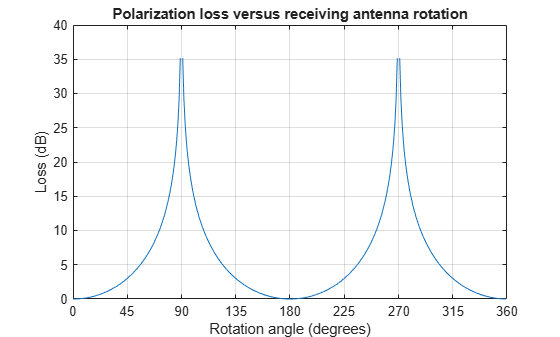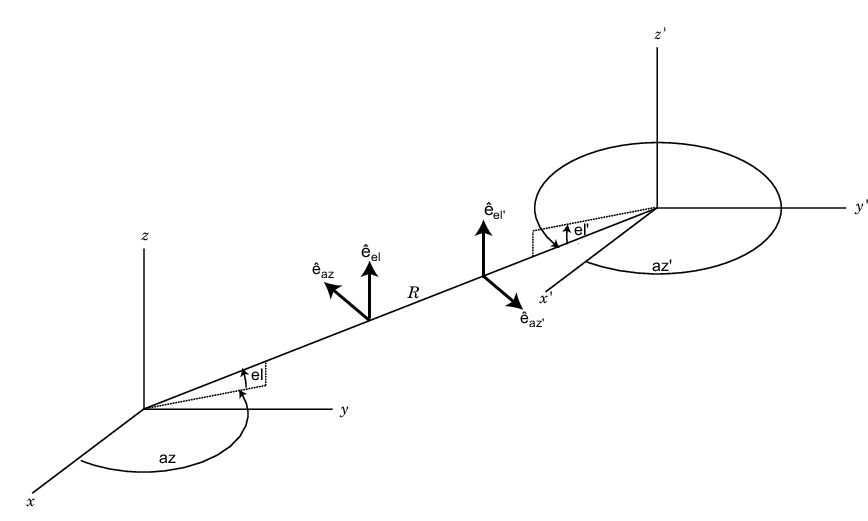# polloss

Polarization loss

## Syntax

``rho = polloss(fv_tr,fv_rcv)``
``rho = polloss(fv_tr,fv_rcv,pos_rcv)``
``rho = polloss(fv_tr,fv_rcv,pos_rcv,axes_rcv)``
``rho = polloss(fv_tr,fv_rcv,pos_rcv,axes_rcv,pos_tr)``
``rho = polloss(fv_tr,fv_rcv,pos_rcv,axes_rcv,pos_tr,axes_tr)``

## Description

example

````rho = polloss(fv_tr,fv_rcv)` returns the loss, in decibels, because of mismatch between the polarization of a transmitted field, `fv_tr`, and the polarization of the receiving antenna, `fv_rcv`. The field vector lies in a plane orthogonal to the direction of propagation from the transmitter to the receiver. The transmitted field is represented as a 2-by-1 column vector `[Eh;Ev]`. In this vector, `Eh` and `Ev` are the field’s horizontal and vertical linear polarization components with respect to the transmitter’s local coordinate system. The receiving antenna’s polarization is specified by a 2-by-1 column vector, `fv_rcv`. You can also specify this polarization in the form of `[Eh;Ev]` with respect to the receiving antenna’s local coordinate system. In this syntax, both local coordinate axes align with the global coordinate system.```

example

````rho = polloss(fv_tr,fv_rcv,pos_rcv)` specifies, in addition, the position of the receiver. The receiver is defined as a 3-by-1 column vector, `[x;y;z]`, with respect to the global coordinate system (position units are in meters). This syntax can use any of the input arguments in the previous syntax.```

example

````rho = polloss(fv_tr,fv_rcv,pos_rcv,axes_rcv)` specifies, in addition, the orthonormal axes, `axes_rcv`. These axes define the receiver's local coordinate system as a 3-by-3 matrix. The first column gives the x-axis of the local system with respect to the global coordinate system. The second and third columns give the y and z axes, respectively. This syntax can use any of the input arguments in the previous syntaxes.```

example

``` `rho = polloss(fv_tr,fv_rcv,pos_rcv,axes_rcv,pos_tr)` specifies, in addition, the position of the transmitter as a 3-by-1 column vector, `[x;y;z]`, with respect to the global coordinate system (position units are in meters). This syntax can use any of the input arguments in the previous syntaxes.```

example

````rho = polloss(fv_tr,fv_rcv,pos_rcv,axes_rcv,pos_tr,axes_tr)` specifies, in addition, the orthonormal axes, `axes_tr`. These axes define the transmitter's local coordinate system as a 3-by-3 matrix. The first column gives the x-axis of the local system with respect to the global coordinate system. The second and third columns give the y and z axes, respectively. This syntax can use any of the input arguments in the previous syntaxes.```

## Examples

collapse all

Begin with a 45° polarized transmitted field and a receiver that is horizontally polarized. By default, the transmitter and receiver local axes coincide with the global coordinate system. Compute the polarization loss in dB.

```fv_tr = [1;1]; fv_rcv = [1;0]; rho = polloss(fv_tr,fv_rcv)```
```rho = 3.0103 ```

The loss is 3 dB as expected because only half the power of the field matches to the receive antenna polarization.

Begin with identical transmitter and receiver polarizations. Place the receiver at a position 100 meters along the y-axis. The transmitter is at the origin (its default position) and both local coordinate axes coincide with the global coordinate system (by default). First, compute the polarization loss. Then, move the receiver 100 meters along the_x_-axis, and compute the polarization loss again.

```fv_tr = [1;0]; fv_rcv = [1;0]; pos_rcv = [0;100;0]; rho(1) = polloss(fv_tr,fv_rcv,pos_rcv); pos_rcv = [100;100;0]; rho(2) = polloss(fv_tr,fv_rcv,pos_rcv)```
```rho = 1×2 0 0 ```

No polarization loss occurs at either position. The spherical basis vectors of each antenna are parallel to other antenna and the polarization vectors are the same.

Start with identical transmitter and receiver polarizations. Put the receiver at a position 100 meters along the y-axis. The transmitter is at the origin (default) and both local coordinate axes coincide with the global coordinate system (default). Compute the loss, and then rotate the receiver 30° around the y-axis. This rotation changes the azimuth and elevation of the transmitter with respect to the receiver and, therefore, the direction of polarization.

```fv_tr = [1;0]; fv_rcv = [1;0]; pos_rcv = [0;100;0]; ax_rcv = azelaxes(0,0); rho(1) = polloss(fv_tr,fv_rcv,pos_rcv,ax_rcv); ax_rcv = roty(30)*ax_rcv; rho(2) = polloss(fv_tr,fv_rcv,pos_rcv,ax_rcv)```
```rho = 1×2 0 1.2494 ```

The receiver polarization vector remains unchanged. However, rotating the local coordinate system changes the direction of the field of the receiving antenna polarization with respect to global coordinates. This change results in a 1.2 dB loss.

Start with identical transmitter and receiver polarizations. Put the receiver at a position 100 meters along the_y_-axis. The transmitter is at the origin (default) and both local coordinate axes coincide with the global coordinate system (default). First, compute the polarization loss. Then, move the transmitter 100 meters along the x-axis and 100 meters along the y-axis, and compute the polarization loss again.

```fv_tr = [1;0]; fv_rcv = [1;0]; pos_rcv = [0;100;0]; ax_rcv = azelaxes(0,0); pos_tr = [0;0;0]; rho(1) = polloss(fv_tr,fv_rcv,pos_rcv,ax_rcv,pos_tr); pos_tr = [100;100;0]; rho(2) = polloss(fv_tr,fv_rcv,pos_rcv,ax_rcv,pos_tr)```
```rho = 1×2 0 0 ```

There is no polarization loss at either position because the spherical basis vectors of each antenna are parallel to their counterparts and the polarization vectors are the same.

Specifying identical transmitter and receiver polarizations, plot the loss as the local receiving antenna axes rotate around the $x$-axis.

```fv_tr = [1;0]; fv_rcv = [1;0];```

The position of the transmitting antenna is at the origin and its local axes align with the global coordinate system. The position of the receiving antenna is 100 meters along the global $x$-axis. However, its local $x$-axis points towards the transmitting antenna.

```pos_tr = [0;0;0]; axes_tr = azelaxes(0,0); pos_rcv = [100;0;0]; axes_rcv0 = rotz(180)*azelaxes(0,0);```

Rotate the receiving antenna around its local $x$-axis in one-degree increments. Compute the loss for each angle.

```angles = [0:1:359]; n = size(angles,2); rho = zeros(1,n); % Initialize space for k = 1:n axes_rcv = rotx(angles(k))*axes_rcv0; rho(k) = polloss(fv_tr,fv_rcv,pos_tr,axes_tr,... pos_rcv,axes_rcv); end```

Plot the polarization loss.

```hp = plot(angles,rho); hax = hp.Parent; hax.XLim = [0,360]; xticks = (0:(n-1))*45; hax.XTick = xticks; grid; title('Polarization loss versus receiving antenna rotation') xlabel('Rotation angle (degrees)'); ylabel('Loss (dB)');```The angle-loss plot shows nulls (`Inf` dB) at 90 degrees and 270 degrees where the polarizations are orthogonal.

## Input Arguments

collapse all

The transmitted field vector in linear component representation specified as a 2-by-1, complex-valued column vector `[Eh;Ev]`. In this vector, `Eh` and `Ev` are the field’s horizontal and vertical linear components.

Example: [1;1]

Data Types: `double`
Complex Number Support: Yes

Receiver polarization vector in linear component representation specified as a 2-by-1, complex-valued column vector `[Eh;Ev]`. In this vector, `Eh` and `Ev` are the polarization vector’s horizontal and vertical linear components.

Example: [0;1]

Data Types: `double`
Complex Number Support: Yes

Receiving antenna position specified as a 3-by-1, real-valued column vector. The components of `pos_rcv` are specified in the global coordinate system as `[x;y;z]`.

Example: [1000;0;0]

Data Types: `double`

Receiving antenna local coordinate axes specified as a 3-by-3, real-valued matrix. Each column is a unit vector specifying the local coordinate system's orthonormal x, y, and z axes, respectively, with respect to the global coordinate system. Each column is written in `[x;y;z]` form. If `axes_rcv` is specified as the identity matrix, the local coordinate system is aligned with the global coordinate system.

Example: [1, 0, 0; 0, 1, 0; 0, 0 ,1]

Data Types: `double`

Transmitter position specified as a 3-by-1, real-valued column vector. The components of `pos_tr` are specified in the global coordinate system as `[x;y;z]`.

Example: [0;0;0]

Data Types: `double`

Transmitting antenna local coordinate axes specified as a 3-by-3, real-valued matrix. Each column is a unit vector specifying the local coordinate system's orthonormal x, y, and z axes, respectively, with respect to the global coordinate system. Each column is written in `[x;y;z]` form. If `axes_tr` is the identity matrix, the local coordinate system is aligned with the global coordinate system.

Example: [1, 0, 0; 0, 1, 0; 0, 0 ,1]

Data Types: `double`

## Output Arguments

collapse all

Polarization loss returned as scalar in decibel units. The polarization loss is the projection of the normalized transmitted field vector into the normalized receiving antenna polarization vector. Its value lies between zero and unity. When converted into dB, (and a sign changed to show loss as positive) its value lies between 0 and `-Inf`.

collapse all

### Polarization Loss Due to Field and Receiver Mismatch

Loss occurs when a receiver is not matched to the polarization of an incident electromagnetic field.

In the case of the polarization of a field emitted by a transmitting antenna, first, look at the far zone of the transmitting antenna, as shown in the following figure. At this location―which is the location of the receiving antenna―the electromagnetic field is orthogonal to the direction from transmitter to receiver.

You can represent the transmitted electromagnetic field, `fv_tr`, by the components of a vector with respect to a spherical basis of the transmitter’s local coordinate system. The orientation of this basis depends on its direction from the origin. The direction is specified by the azimuth and elevation of the receiving antenna with respect to the transmitter’s local coordinate system. Then, the transmitter’s polarization, in terms of the spherical basis vectors of the transmitter’s local coordinate system, is

`$E={E}_{H}{\stackrel{^}{e}}_{az}+{E}_{V}{\stackrel{^}{e}}_{el}={E}_{m}{P}_{i}$`

In the same manner, the receiver’s polarization vector, `fv_rcv`, is defined with respect to a spherical basis in the receiver’s local coordinate system. Now, the azimuth and elevation specify the transmitter’s position with respect to the receiver’s local coordinate system. You can write the receiving antennas polarization in terms of the spherical basis vectors of the receiver’s local coordinate system:

`$P={P}_{H}{{\stackrel{^}{e}}^{\prime }}_{az}+{P}_{V}{{\stackrel{^}{e}}^{\prime }}_{el}$`

This figure shows the construction of the different transmitter and receiver local coordinate systems. It also shows the spherical basis vectors with which to write the field components.The polarization loss is the projection (or dot product) of the normalized transmitted field vector onto the normalized receiver polarization vector. Notice that the loss occurs because of the mismatch in direction of the two vectors not in their magnitudes. Because the vectors are defined in different coordinate systems, they must be converted to the global coordinate system in order to form the projection. The polarization loss is defined by:

`$\rho =\frac{|{E}_{i}\cdot P{|}^{2}}{|{E}_{i}{|}^{2}|P{|}^{2}}$`

 Mott, H. Antennas for Radar and Communications.John Wiley & Sons, 1992.